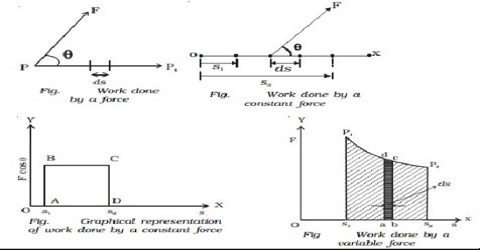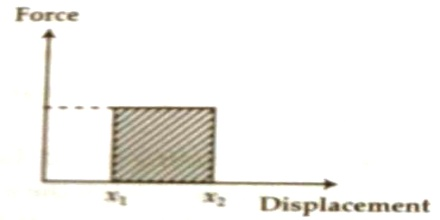# Graphical Representation of Work

Graphical representation of work

A graph is drawn by taking component of applied force along y-axis and displacement along x-axis {Fig (a)]. Area underneath this graph is equal to work done. If the applied force is constant then the graph will be a straight line parallel to X-axis. Suppose, due to the action of this force the body moves from position x1 to position x2. Then the volume of work done from the graph is obtained from the area of the rectangle subtended by the force in the Y-axis and difference x2 – x1 in the x-axis. In the figure, it has been shown by rectangle drawn by dark lines.If the applied force is variable, then the graph can be of any shape [Fig.b]. Here also work done can be determined by the previous method. For exceedingly small displacement ∆x force F may be considered constant. So, for that small displacement work done is F∆x. This work is equal to the area of the strip drawn in the X-axis. We can Force consider the displacement from x1 to x2 as the summation composed of innumerable similar strips ∆x. For each of the strips work done can be determined from the graph which is equal to F∆x and by summing all these small works we can get total work done. So, as above, work done for the small strip is the area of the rectangle drawn between X-axis and two ordinates bounded by x1 and x2.That means, when there is displacement of a body due to the application of force on it, then work is done. Work is measured by the product of applied force on the body and the displacement along the direction of force.

That means, work = applied force x displacement of the point of action of the force along the direction of the force.

so, W = Fs

Lf the displacement is alone the direction making an angle θ with the applied force, then work = force x component of displacement along the direction of force.

so, W = Fs cosθ

Unit: S.I. unit of work is Joule or Newton-Metre (N-m).Work is a scalar quantity. Due to application of 1 Newton of force on a body if the displacement is 1 metre, then work done is called 1 Joule.